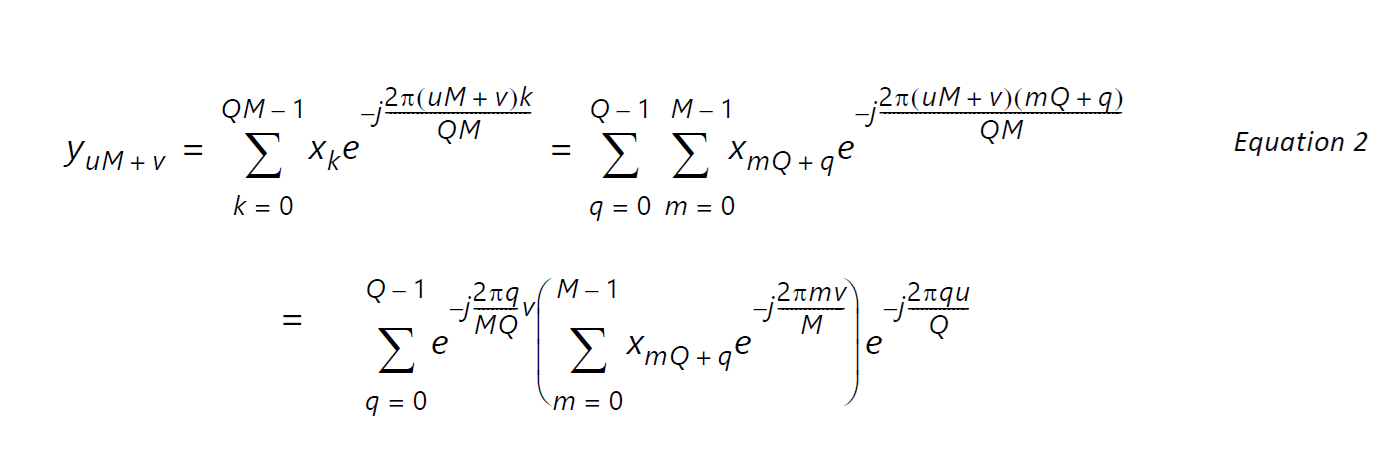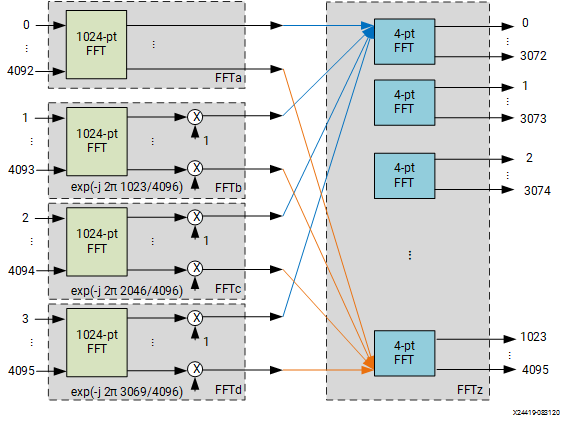# FFT on Multiple AI Engines

## Block-by-Block Configurable Fast Fourier Transform Implementation on AI Engine (XAPP1356)

Document ID
XAPP1356
Release Date
2021-01-11
Revision
1.0 English

Given an FFT size N = Q M, the N-point FFT it can be partitioned into Q FFTs of M points, followed by a linear phase rotation, and then M FFTs of Q points to obtain the results. More specifically, Equation 1 can be re-written as follows.It is observed that the summation inside the bracket is an M-point FFT on the input data and the summation outside is a Q-point FFT; between them there is a linear phase rotation with a fixed phase increment of -2π q/N, where q is the phase index.

Every AI Engine has access to four memory groups so a natural choice of Q is 4. For N=4096, the FFT input is divided into four phases going into four AI Engines in parallel. The AI Engines perform 1024-point FFT on the data followed by a linear phase rotation before the data are output to the fifth AI Engine for 4-point FFTs. This process is illustrated in the following figure where every grey rectangle shows the functionalities implemented in each AI Engine, called FFTa, FFTb, FFTc, FFTd, and FFTz, respectively.

Figure 1. Partitioning 4096-point FFT onto Five AI EnginesBesides 4096-point FFT, the proposed five AI Engine architecture supports all the modes listed in Table 1 by using if-else conditional branches in the AI Engine kernels. The 1024-point FFT kernels perform either 512-point or 1024-point FFT/IFFTs according to the control word, and the fifth AI Engine, FFTz, performs four-point FFT/IFFT in 4096-point mode, two-point FFT/IFFT in 2048-point mode, and a direct memory copy in 512 or 1024-point modes.